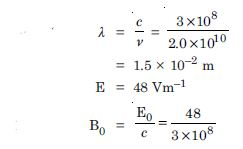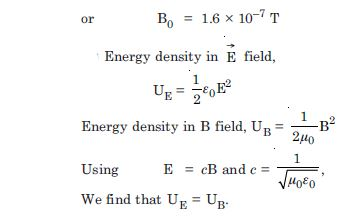# NCERT Solutions for Class 12 Physics Chapter 2 - Electromagnetic Waves

Get a thorough understanding of the chapter “Electromagnetic Waves” with the help of NCERT Solutions on Aasoka, the top eLearning platform. Students can start their learning process in order to score the marks they desire in their board exams. The questions of NCERT Solutions for Class 12th is designed are as per the latest syllabus and provide an understanding of the chapter. Students can get a good grasp on the topics of the chapter of Physics Class 12th.

“Electromagnetic Waves” chapter of Class 12th Physics includes the explanation of the topic of magnetism, capacitor, electromagnetism, Kirchhoff’s rules, potential difference and displacement, RMS value of conduction current, and similarities between displacement and conduction current. Take the help of NCERT Solutions to understand each concept efficiently and effectively.

##### Question 1:

Fig. TBQ 8.1 shows a capacitor made of two circular plates each of radius 12 cm, and separated by 5.0 cm. The capacitor is being charged by an external source (not shown in the figure). The charging current is constant and equal to 0.15 A.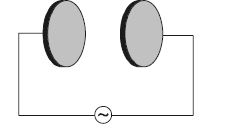Calculate the capacitance and rate of change of potential difference between the plates. Obtain the displacement current across the plates.

Is Kirchhoff’s first rule (junction rule) valid at each plate of the capacitor ? Explain.

r = 0.12 m,
d = 5.0 cm = 5 × 10–2 m,
I = 0.15 A
Capacitance of the capacitor,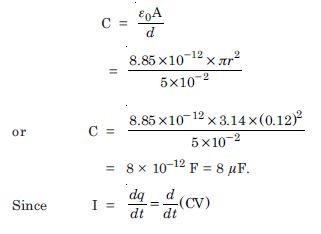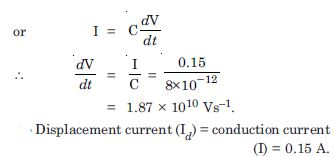Yes, Kirchhoff’s first law holds good at each plate if we take both conduction and displacement current. The conduction current arriving at a plate is equal to the displacement current leaving that plate.

##### Question 2:

A parallel plate capacitor (Fig. TBQ 8.2) made of circular plates each of radius R = 6.0 cm has a capacitance C = 100 pF. The capacitor is connected to a 230 V a.c. supply with a (angular) frequency of 300 rad–1 s.

What is r.m.s. value of conduction current ?

Is the conduction current equal to the displacement current ?

Determine the amplitude of B at a point 3.0 cm from the axis between the plates.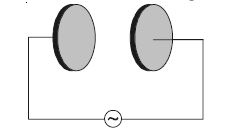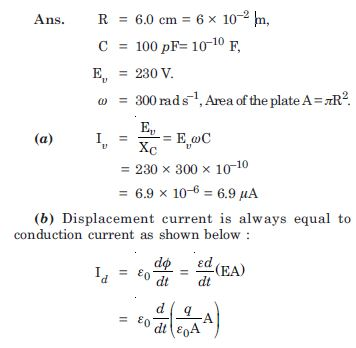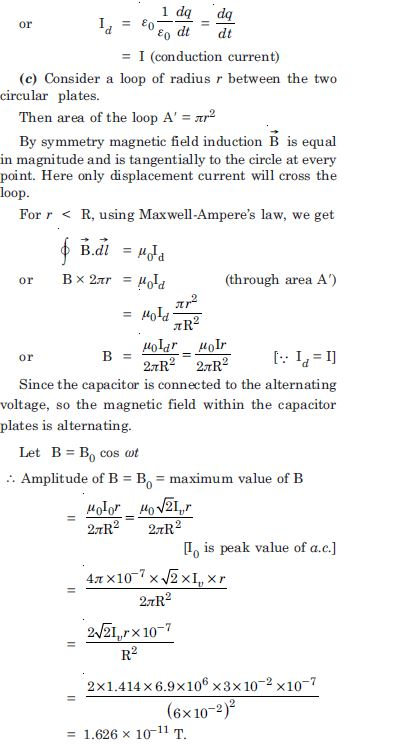##### Question 3:

What physical quantity is the same for X-rays of wavelength 10–10 m, red light of wavelength 6800 Å and radio waves of wavelength 500 m ?

The speed in vacuum is the same for all.
c = 3 × 108 ms–1 .

##### Question 4:

A plane electromagnetic wave travels in vacuum along z-direction. What can you say about the direction of its electric and magnetic field vectors ? If the frequency of the wave is 30 MHz, what is its wavelength ?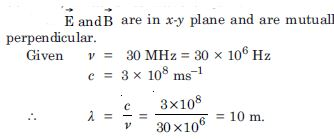##### Question 5:

A radio can tune in to any station in the 7.5 MHz to 12 MHz band. What is the corresponding wavelength band ?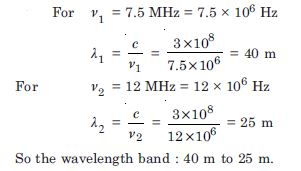##### Question 6:

A charged particle oscillates about its mean equilibrium position with a frequency of 109 Hz. What is the frequency of the electromagnetic waves produced by the oscillator ?

The frequency of e.m. waves produced by the oscillator is equal to the frequency of oscillation of the charged particle about its mean position i.e. 109 Hz.

##### Question 7:

The amplitude of the magnetic field part of a harmonic electromagnetic wave in vacuum is B0 = 510 nT. What is the amplitude of the electric field part of the wave ?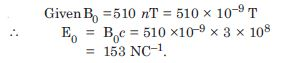##### Question 8: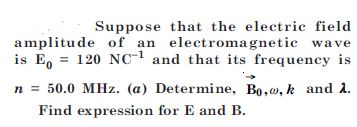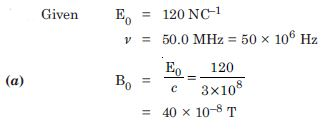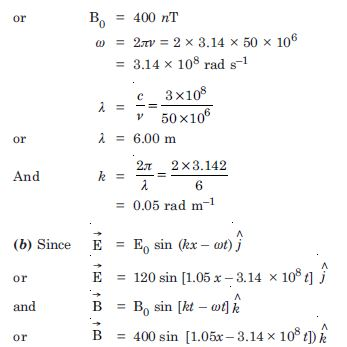##### Question 9:

The terminology for different parts of the electromagnetic spectrum is given in the text, use the formula E = h$\mathrm{\nu }$ (for energy of a quantum of radiation : photon) and obtain the photon energy in units of eV for different parts of the e.m. spectrum. In what way are the different scales of photon energies that you obtain related to the sources of electromagnetic radiation ?

Photon energy for $\mathrm{\lambda }$ = 1m is,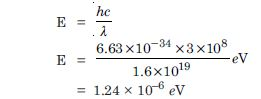Photon energy for other wavelength in the figure for e.m. spectrum can be obtained by multiplying appropriate powers of ten. Energy of a photon that a source produces indicates the spacing of the relevant energy levels of the sources. For example $\mathrm{\lambda }$ = 1.0–12 m corresponds to photon energy = 1.24 × 106 eV = 1.24 MeV. This indicates that nuclear energy levels (transition between which cause γ-ray emission) are typically spaced by 1 MeV or so. Similarly, a visible wavelength $\mathrm{\lambda }$ = 2 × 10–7 m corresponds to photon energy = 2.5 eV. This implies that energy levels (transitions between which give visible radiation) are typically spaced by a few eV.

##### Question 10:

In a plane e.m. wave, the electric field oscillates sinusoidally at a frequency of 2.0 × 1010 Hz and amplitude 48 Vm–1.
What is the wavelength of the wave ?
What is the amplitude of the oscillating magnetic field ?
Show that the average energy density of the E field equals to the average energy density of the B field.[c = 3.0 × 108 ms –1 ]